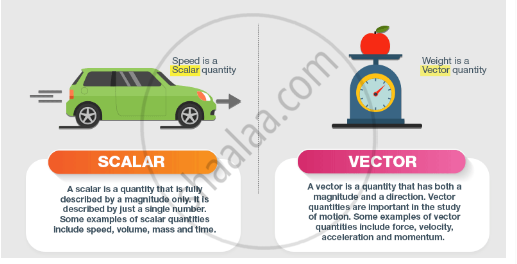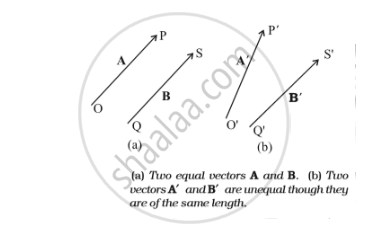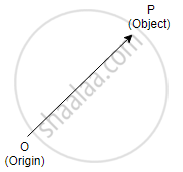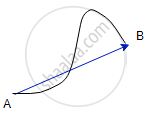# Scalars and Vectors

• Scalars
• Vectors
• Position vector
• Displacement vector
• Resultant vector

## Scalars and Vectors

Mathematics and Science were invented by humans to understand and describe the world around us. A lot of mathematical quantities are used in Physics to explain the concepts clearly. A few examples of these include force, speed, velocity and work. These quantities are often described as being a scalar or a vector quantity. Scalars and vectors are differentiated depending on their definition.

A scalar quantity is defined as the physical quantity that has only magnitude, for example, length, speed, work, mass, density, etc.
On the other hand, a vector quantity is defined as the physical quantity that has both, magnitude as well as direction like displacement, force, torque, momentum, acceleration, velocity, etc.
The other way of differentiating these two quantities is by using a notation.Characteristics of Vectors

• These possess both magnitude and direction.

• These do not obey the ordinary law of algebra.

• These change if either magnitude or direction or both change.

• These are represented by bold-faced letters having arrow over them.

Equality of Vectors
Two vectors A and B are said to be equal if, and only if, they have the same magnitude and the same direction. Figure below shows two equal vectors A and B. We can easily check their equality. Shift B parallel to itself until its tail Q coincides with that of A, i.e. Q coincides with O. Then, since their tips S and P also coincide, the two vectors are said to be equal. In general, equality is indicatedas A = B. Note that in Fig.(b) above, vectors A′ and B′ have the same magnitude but they are not equal because they have different directions. Even if we shift B′ parallel to itself so that its tail Q′ coincides with the tail O′ of A′ , the tip S′ of B′ does not coincide with the tip P′ of A′ .

## Position And Displacement Vectors

One of the primary things to start with Kinematics is to know what position vector is, what displacement vector is, and what the difference between position vector and displacement vector is.

Position Vector:
Position vector is used to specify the position of a certain body. Knowing the position of a body is vital when it comes to describe the motion of that body. The position vector of an object is measured from the origin, in general. Suppose an object is placed in the space as shown below:Position vector(bar r)=xî+ yhat j + zhat k
Where,
î = unit vector along x direction
hat j = unit vector along y direction
hat k = unit vector along z direction

So if an object is at a certain point P (say) at a certain time, its position vector is given as described above.

Displacement Vector:
The displacement vector is a vector which gives the position of a point with reference to a point other than the origin of the co-ordinate system.

The change in the position vector of an object is known as displacement vector. Suppose an object is at point A at time = 0 and at point B at time = t. The position vectors of the object at point A and at point B are given as:
Position vector at A =hat r_A=5hat i+3 hat j + 4 hat k
Position vector at B =hat r_B=2 hat i+2 hat j + 1 hat k

Now, the displacement vector of the object from time interval 0 to t will be:
hat r_B - hat r_A= -3 hat i - hat j - 3 hat k

The displacement of an object can also be defined as the vector distance between the initial point and the final point. Suppose an object travels from point A to point B in the path shown in the black curve belowThe displacement of the particle would be the vector line AB, headed in the direction A to B. The direction of displacement vector is always headed from initial point to the final point.

If you would like to contribute notes or other learning material, please submit them using the button below.

### Shaalaa.com

Motion in plane part 1 (Introduction to Scalar and Vector) [00:10:32]
S